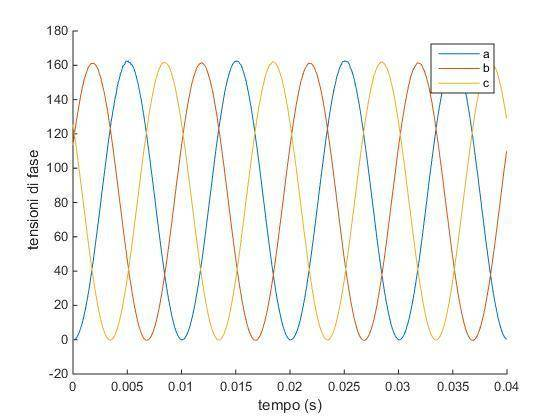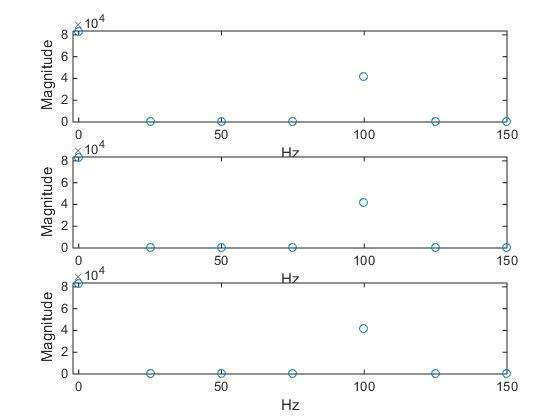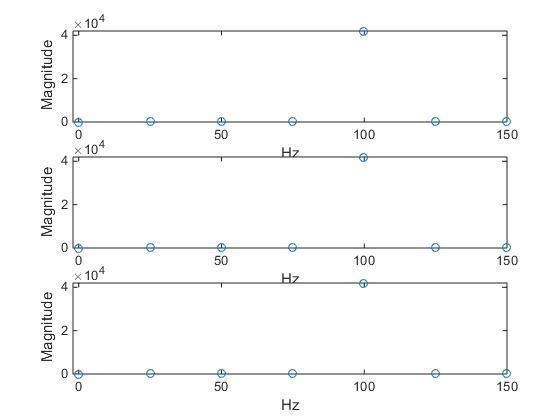# Matlab - calculate phase shift using fft

hello,

I have 3 signals in the form of sampled values. When I plot them using

plot (t,vPa,t,vPb,t,vPc)

where vPa, vPb, vPc contains the values and t contains the sampling istants I get this:when I calculate phase shift using fft I get phase angle = 0.
I have tried using my own code ( which works normally on other vectors) and also a function downloaded from Mathworks and the result is always zero.

The mathworks function is at the end.

what am I doing wrong?

Thanks
Mattia

Matlab:
%%%%%%%%%%%%%%%%%%%%%%%%%%%%%%%%%%%%%%%%%%%%%%%%%%%%%%%%
%              Phase Difference Measurement            %
%               with MATLAB Implementation             %
%                                                      %
% Author: M.Sc. Eng. Hristo Zhivomirov        12/01/14 %
%%%%%%%%%%%%%%%%%%%%%%%%%%%%%%%%%%%%%%%%%%%%%%%%%%%%%%%%

function PhDiff = phdiffmeasure(x, y)

% function: PhDiff = phdiffmeasure(x, y)
% x - first signal in the time domain
% y - second signal in the time domain
% PhDiff - phase difference Y -> X, degrees

% represent x as column-vector if it is not
if size(x, 2) > 1
x = x';
end

% represent y as column-vector if it is not
if size(y, 2) > 1
y = y';
end

% signals length
xlen = length(x);
ylen = length(y);

% window preparation
xwin = hanning(xlen, 'periodic');
ywin = hanning(ylen, 'periodic');

% fft of the first signal
X = fft(x.*xwin);

% fft of the second signal
Y = fft(y.*ywin);

% phase difference calculation
[~, indx] = max(abs(X));
[~, indy] = max(abs(Y));
PhDiff = angle(Y(indy)) - angle(X(indx));
PhDiff = PhDiff*180/pi;

end

## Answers and Replies

jedishrfu
Mentor
I don't see what you did wrong.

However, have you looked at the intermediate values for X and Y and the angle(X(indx)) values that are used to compute the phase difference?

I have now, angle(Y(indy)) and angle(X(indx)) are both zero.

I have attached the original 3 vectors.

#### Attachments

• vectors.txt
26.1 KB · Views: 589
Last edited:
I have found a different way to calculate phase shift that can be used in this case:

h1 = hilbert(vPa);

h2 = hilbert(vPb);

phase_rad = max(angle(h1 ./ h2))

which gives back the correct value of 2/3 * pi.

However I would be couriois to know why for this specific case fft didnt work. I thought it was a pretty generic method

jedishrfu
Mentor
I meant the X and Y in the function you showed.

Aren't they supposed to be mostly zeros with a few spikes?

I think you need to look at each line of the function to decide if its doing the right thing.

The code looks like it applies an FFT to the two input signals to produce a frequency spectrum. From that it determines the highest spike and from that subtracts them to determine the phase difference.

yes that is what it does. Also my own code did that. ffts are mostly zero with one big spike on 50 Hz.
I am positive both my own code and the one I posted are correct. becaouse I have used them many times and they returned correct results

jedishrfu
Mentor
Then I guess there's something unique about your input data and the function you listed. Perhaps if you step through the function interactively you'll be able to spot the difference. It could be a number of elements limit or datatype mismatch...

jedishrfu
Mentor
Perhaps your data doesn't have an FFT with a significant frequency that can be picked out i.e. no one max value

Try plotting the FFT's of both signals to see what you get.

•daymos
thanks for the spectrum plot tips jedishrfu
I haven't solved it yes but I think you pointed me in the right direction.

this is the spectrum of the 3 signals:So there is a huge spike at zero Hz.

I have composed each signal multiplying 2 previous signals that where at 50Hz. So I expect the new signals to be at 100 Hz. And I see a spike there.
But I dont understand why the spike in zero.

Last edited:
You have a DC component in you signal. max function should detected the peak of the periodic signal ?

You have a DC component in you signal. max function should detected the peak of the periodic signal ?

when I use max function it gives back the index of frequency = 0 HZ

Then the answer is correct, the DC components have zero phase shift :)Then the answer is correct, the DC components have zero phase shift :)

well, ok thats correct.

Since I need the 100Hz I subtracted the mean value of the signal to itself.

thanks a lot!

The zero value of the fourier transform will always just be the mean give a or take a factor of pi and volume.

boneh3ad
Science Advisor
Gold Member
In general, it's usually a good idea to make sure the signal you wish to pass through your FFT has zero mean, that way you are only dealing with nonzero frequency components.

the original 2 signals had no DC component. But by multiplying them value by value the resulting signal was no longer centered on the zero for the vertical axis.
Pretty obvious after you get it..

what did you change ad your coding to make that output? thanks in advance :)